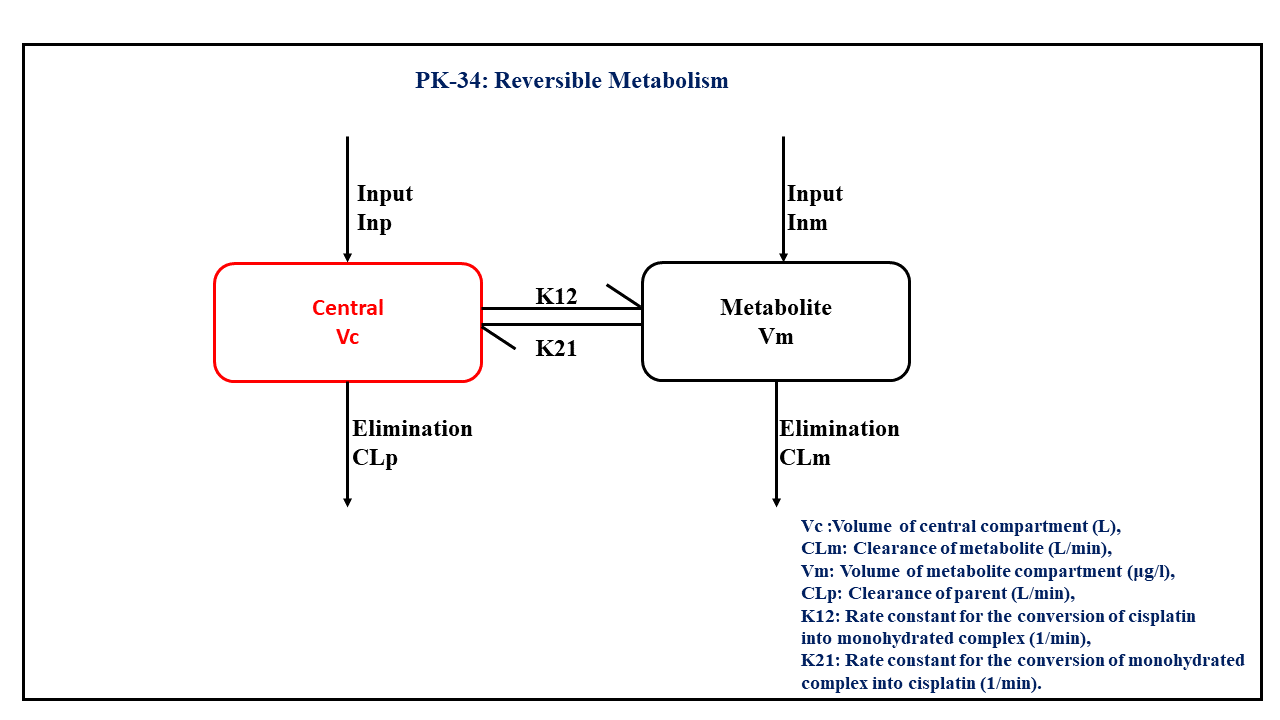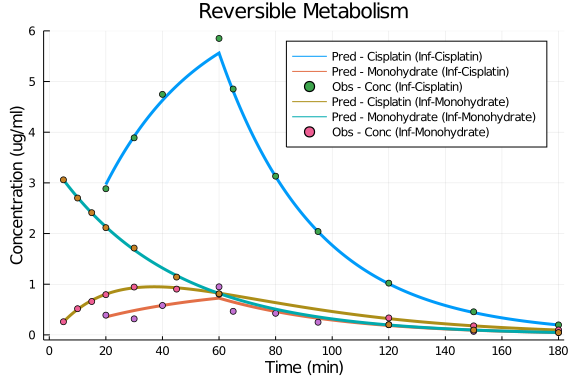# Exercise PK34 - Reversible Metabolism

### Background

• Structural model - Two compartment model

• Route of administration - IV-infusion (with an infusion pump)

• Dosage Regimen - 100 mg/m² of Cisplatin for 1-h at time=0 considering a patient with 1.7m²

• Number of Subjects - 1### Learning Outcome

• By the application of the present model, we will learn how to simulate model for reversible metabolism.

• Simulate data for two IV-Infusion with two different rates of infusion regimen and how to develop a better fit for the available data.

### Objectives

In this exercise you will learn how to

• Simulate an IV-infusion two compartment for reversible metabolism.

(Here certain assumptions to be considered:-

• A fraction of the dose (2.3%) is present as the monohydrated complex in the infusion solution,

• That there is a reversible reactions between cisplatin (p) and its monohydrated complex (m),

• The input rate can be split into cisplatin infusion rate (Inp) and monohydrate infusion rate (Inm).

• Write a differential equation for a two-compartment model in terms of Clearance and Volume for simulating reversible metabolism.

### Libraries

call the "necessary" libraries to get start.

using Pumas
using Plots
using CSV
using StatsPlots
using Random


### Model - Microconstant Model

In this two compartment model, we administer the mentioned dose on Central compartment as well as on Metabolite compartment at 'time= 0'. Also, K12 and K21 are the rate constants for the conversion of cisplatin into monohydrated complex and the monohydrated complex into cisplatin, respectively.

pk_34             = @model begin
@param begin
tvvc        ∈ RealDomain(lower=0)
tvclm       ∈ RealDomain(lower=0)
tvvm        ∈ RealDomain(lower=0)
tvclp       ∈ RealDomain(lower=0)
tvk12       ∈ RealDomain(lower=0)
tvk21       ∈ RealDomain(lower=0)
Ω           ∈ PDiagDomain(6)
σ²_prop     ∈ RealDomain(lower=0)
end

@random begin
η           ~ MvNormal(Ω)
end

@pre begin
Vc          = tvvc * exp(η)
CLm         = tvclm * exp(η)
Vm          = tvvm * exp(η)
CLp         = tvclp * exp(η)
K12         = tvk12 * exp(η)
K21         = tvk21 * exp(η)
end

@dynamics begin
Central'    = -(CLp/Vc)*Central - K12*Central + K21*Metabolite*Vc/Vm
Metabolite' = -(CLm/Vm)*Metabolite - K21*Metabolite + K12*Central*Vm/Vc
end

@derived begin
cp          = @. Central/Vc
dv_cp       ~ @. Normal(cp, sqrt(cp^2*σ²_prop))
met         = @. Metabolite/Vm
dv_met      ~ @. Normal(met, sqrt(cp^2*σ²_prop))
end
end

PumasModel
Parameters: tvvc, tvclm, tvvm, tvclp, tvk12, tvk21, Ω, σ²_prop
Random effects: η
Covariates:
Dynamical variables: Central, Metabolite
Derived: cp, dv_cp, met, dv_met
Observed: cp, dv_cp, met, dv_met


### Parameters

Parameters provided for simulation are as below. tv represents the typical value for parameters.

• Vc - Volume of central compartment (L)

• CLm - Clearance of metabolite (L/min)

• Vm - Volume of metabolite compartment (μg/L)

• CLp - Clearance of parent (L/min)

• K12 - Rate constant for the conversion of cisplatin into monohydrated complex (min⁻¹)

• K21 - Rate constant for the conversion of monohydrated complex into cisplatin (min⁻¹)

• Ω - Between Subject Variability

• σ - Residual error

param = ( tvvc    = 14.1175,
tvclm   = 0.00832616,
tvvm    = 2.96699,
tvclp   = 0.445716,
tvk12   = 0.00021865,
tvk21   = 0.021313,
Ω       = Diagonal([0.0,0.0,0.0,0.0,0.0,0.0]),
σ²_prop = 0.001)

(tvvc = 14.1175, tvclm = 0.00832616, tvvm = 2.96699, tvclp = 0.445716, tvk1
2 = 0.00021865, tvk21 = 0.021313, Ω = [0.0 0.0 … 0.0 0.0; 0.0 0.0 … 0.0 0.0
; … ; 0.0 0.0 … 0.0 0.0; 0.0 0.0 … 0.0 0.0], σ²_prop = 0.001)


### Dosage Regimen

In this section the Dosage regimen is mentioned for:

• Cisplatin Infusion - A total dose of 170mg (100mg/m² * 1.7m²) i split as Cisplatin 166.09 and Monohydrate 3.91.

• Monohydrate Infusion - A total dose of 10 mg/L is given as Monohydrate

ev1  = DosageRegimen([166.09,3.91], time=0, cmt=[1,2], duration=[60,60])
sub1 = Subject(id=1, events=ev1, time=20:0.1:180)
ev2  = DosageRegimen(10, time=0, cmt=2, duration=2)
sub2 = Subject(id=2, events=ev2, time=5:0.1:180)
pop2_sub = [sub1, sub2]

Population
Subjects: 2
Covariates:


### Simulation

We will simulate the plasma concentration at the pre specified time points.

Random.seed!(123)
sim_sub1 = simobs(pk_34, pop2_sub, param)
df1      = DataFrame(sim_sub1)


### Dataframe and Plot

Convert the simulation to a dataframe and use the dataframe to make your necessary plots

df1_id1    = filter(x -> x.id == "1", df1)
df1_id1_dv = filter(x -> x.time in [20,30,40,60,65,80,95,120,150,180], df1_id1)
df2_id2    = filter(x -> x.id == "2", df1)
df2_id2_dv = filter(x -> x.time in [5,10,15,20,30,45,60,120,150,180], df2_id2)

@df df1_id1 plot(:time, :cp,
title="Reversible Metabolism" , label="Pred - Cisplatin (Inf-Cisplatin)",
xlabel="Time (min)", ylabel="Concentration (ug/ml)", linewidth=3,
xticks=[0,20,40,60,80,100,120,140,160,180], xlims=(-2,182), yticks=[0,1,2,3,4,5,6], ylims=(-0.1,6))
@df df1_id1 plot!(:time, :met, label="Pred - Monohydrate (Inf-Cisplatin)", linewidth=3)
@df df1_id1_dv scatter!(:time, :dv_cp, label="Obs - Conc (Inf-Cisplatin)")
@df df1_id1_dv scatter!(:time, :dv_met, label=false)

@df df2_id2  plot!(:time, :cp, label="Pred - Cisplatin (Inf-Monohydrate)", linewidth=3)
@df df2_id2  plot!(:time, :met, label="Pred - Monohydrate (Inf-Monohydrate)", linewidth=3)
@df df2_id2_dv scatter!(:time, :dv_cp, label="Obs - Conc (Inf-Monohydrate)")
@df df2_id2_dv scatter!(:time, :dv_met, label=false)